图像处理的线性变换c程序_图像处理,图像的非线性变换 - CSDN
• 图像灰度线性变换 假定原图像f(x,y)f(x,y)f(x,y)的灰度范围为[a,b]，变换后图像g(x,y)g(x,y)g(x,y)灰度扩展为[c,d],则根据线性方程式可以得到： (9-1)g(x,y)=d−cb−a[f(x,y)−a]+c g(x,y) = \frac{d - c}{b - a}[f...
图像灰度线性变换
假定原图像$f(x,y)$的灰度范围为[a,b]，变换后图像$g(x,y)$灰度扩展为[c,d],则根据线性方程式可以得到：
$g(x,y) = \frac{d - c}{b - a}[f(x,y) - a] + c \tag{9-1}$
通过上式可以把图像某个亮度值区域[a,b]扩展到[c,d]。采用等例线性灰度变换对图像每一个像素做线性灰度拉伸，将有效地改善图像效果。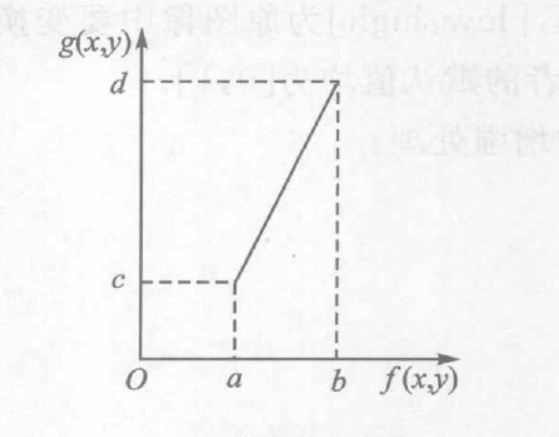若图像灰度在$0-M$范围内，其中大部分像素的灰度级分布在区间[a,b]内，很小部分像素的灰度级超出此区间，则映射关系为：
$g(x,y) = \begin{cases} c & 0 \leq f(x,y) \leq a \\ \frac{d-c}{b-a}[f(x,y) - a] + c & a \lt f(x,y) \lt b \\ d &b \lt f(x,y) \leq M \end{cases} \tag{9-2}$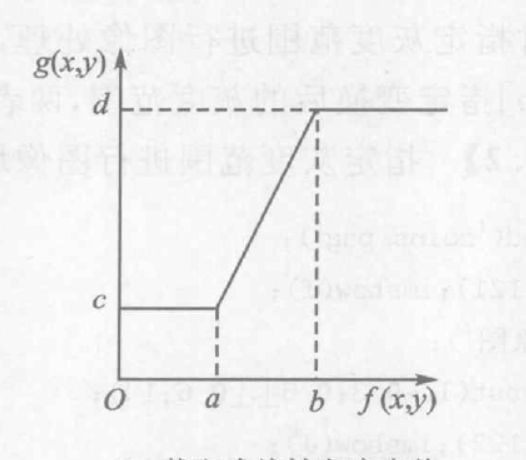Python实现代码如下：
def linear_transform(src,c = 0,low=0,high=1,bottom=0,up=1):
assert high >= low and high >= 0 and high <= 1 and low >= 0 and low <= 1
assert up > bottom and up >= 0 and up <= 1 and bottom >= 0 and bottom <= 1

dst = np.zeros_like(src).astype(np.float32)
dst = (up - bottom) / (high - low) * (src.astype(np.float32)-low) + c
dst = np.clip(dst,0,255).astype(np.uint8)
return dst

程序运行结果左边为原图像，右边为图像灰度线性变换后的结果


展开全文图像灰度变换 图像处理 数字图像处理 人工智能
• 本文详细 介绍-图像线性变换算法及实现，并给出了一份完整程序DEMO下载链接，跟大家分享一下，希望大家喜欢！

图像线性变换即线性点运算，输出灰度级与输入灰度级呈线性关系的点运算。公式如2-(9)所示。其中，K,L为变换参数，k属于[0,5],L属于[-128, 128]。
如果k<l< span="" style="word-wrap: normal;">，则输出图像的对比度将增大，反之对比度将减小，k=1，L=0时，输出图像为输入图像的副本，L是对图像亮度的调整。
[函数代码]
///
/// Linear transform process(f=kf+v).
///
/// Source image.
/// Parameter,from 0 to 5.
/// Parameter,from -128 to 128.
///
public static WriteableBitmap LinearTransformProcess(WriteableBitmap src, double k,int v)8线性变换处理
{
if(src!=null )
{
int w = src.PixelWidth;
int h = src.PixelHeight;
WriteableBitmap linearImage = new WriteableBitmap(w,h);
byte[] temp = src.PixelBuffer.ToArray();
for (int i = 0; i < temp.Length; i +=4)
{
temp[i] = (byte)(((k * temp[i] + v + 0.5) > 255 ? 255 : (k * temp[i] + v + 0.5)) < 0 ? 0 : ((k * temp[i] + v + 0.5) > 255 ? 255 : (k * temp[i] + v + 0.5)));
temp[i+1] = (byte)(((k * temp[i+1] + v + 0.5) > 255 ? 255 : (k * temp[i+1] + v + 0.5)) < 0 ? 0 : ((k * temp[i+1] + v + 0.5) > 255 ? 255 : (k * temp[i+1] + v + 0.5)));
temp[i+2] = (byte)(((k * temp[i+2] + v + 0.5) > 255 ? 255 : (k * temp[i+2] + v + 0.5)) < 0 ? 0 : ((k * temp[i+2] + v + 0.5) > 255 ? 255 : (k * temp[i+2] + v + 0.5)));
}
Stream sTemp = linearImage.PixelBuffer.AsStream();
sTemp.Seek(0, SeekOrigin.Begin);
sTemp.Write(temp, 0, w * 4 * h);
return linearImage;
}
else
{
return null;
}
}
[图像效果]Fig.1原图Fig.2效果图(k=4.0,v=64)


展开全文• 承接上一篇博客，接下来实现以下灰度图像的线性拉伸变换，具体理论就不赘述了，学过数字图像处理的基本都知道，下面来看看如何通过VC6.0的MFC编程实现它。 首先打开工作空间的resources视窗，创建菜单项，如下： ...

承接上一篇博客，接下来实现以下灰度图像的线性拉伸变换，具体理论就不赘述了，学过数字图像处理的基本都知道，下面来看看如何通过VC6.0的MFC编程实现它。
首先打开工作空间的resources视窗，创建菜单项，如下：然后为菜单创建消息响应函数，右键灰度线性变换，选择ClassWizard，创建单击事件函数：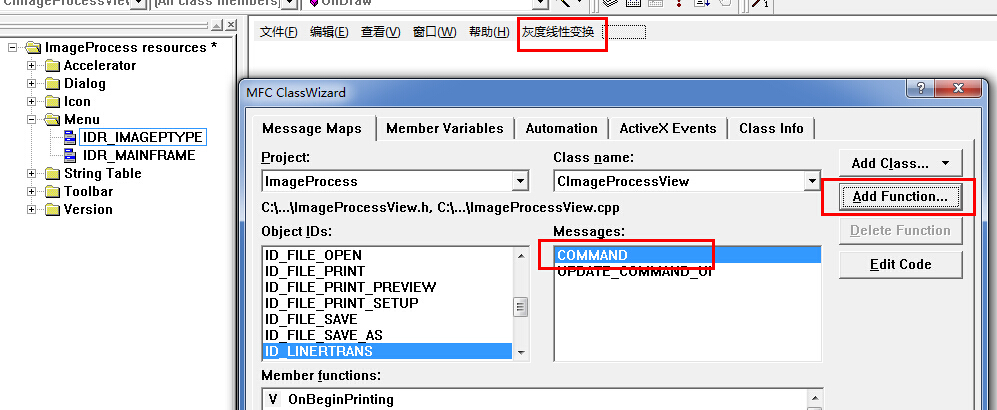确定，点击EditCode后发现有View.cpp中已经有如下代码：

void CImageProcessView::OnLinertrans()
{

}
下面，我们需要创建一个对话框，来接受用户设置的线性变换参数，最小值和最大值，可以右键Dialog，InsertDialog，设置对话框ID为：IDD_DIALOG_LinerTransSet
添加两个Edit编辑框，ID分别为：IDC_EDIT_MAX、IDC_EDIT_MIN,如下：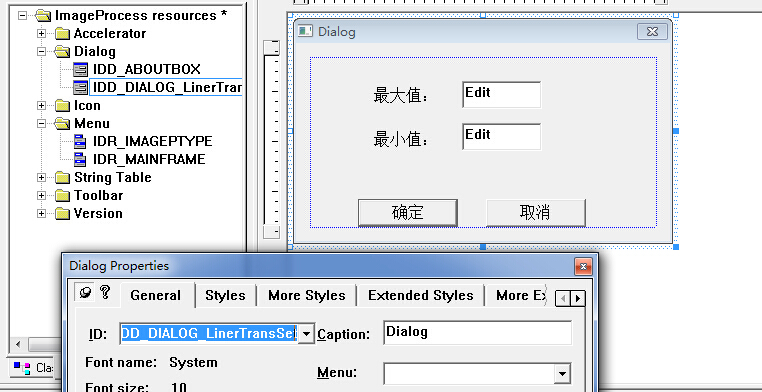为对话框创建类，双击对话框，提示创建新的类，选择确定，设置类参数如下：确定之后，为两个Edit对象创建成员变量，在上面的类向导中，选择Member Variables选项卡，创建成员变量如下：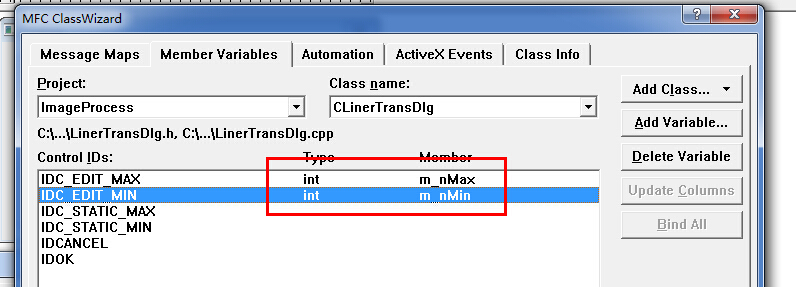这些准备工作做好了之后，便可以实现前面空着的OnLinertrans函数了，先在View.cpp文件上方添加头文件包含# include "LinerTransDlg.h"，然后实现OnLinertrans，
直接上代码如下：
void CImageProcessView::OnLinertrans()
{
CLinerTransDlg linedlg;

if(linedlg.DoModal()==IDOK)
{
UpdateData(TRUE);
CImageProcessDoc* pDoc=GetDocument();
ASSERT_VALID(pDoc);

BYTE* pData=pDoc->m_bmpFile.m_pImgDat;

int cols=pDoc->m_bmpFile.m_Cols;
int rows=pDoc->m_bmpFile.m_Rows;
int min=linedlg.m_nMin;
int max=linedlg.m_nMax;

float mingray=255,maxgray=0;

for(int r=0;r<rows;r++)
{
for(int c=0;c<cols;c++)
{
if(*(pData+cols*r+c)<mingray)
mingray=*(pData+cols*r+c);

if(*(pData+cols*r+c)>maxgray)
maxgray=*(pData+cols*r+c);

}
}
float a=(min-max)/(mingray-maxgray);
for(r=0;r<rows;r++)
{
for(int c=0;c<cols;c++)
{
int gray=int(min+a*(*(pData+cols*r+c)-mingray));
*(pData+cols*r+c)=BYTE(gray);
}
}
Invalidate();
}
}

这样，整个编程任务完成，运行程序：确定后结果如下：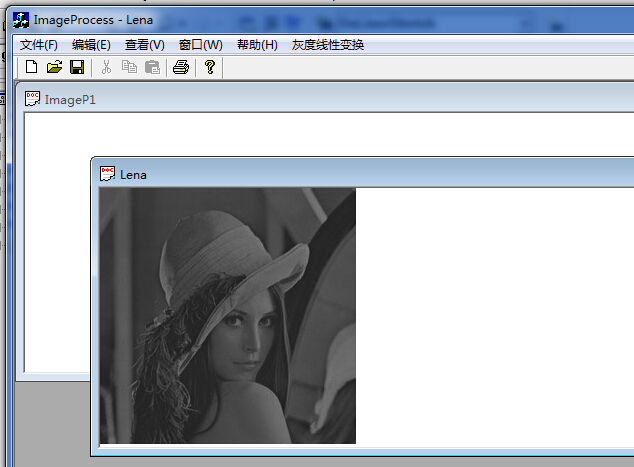写博客不多，欢迎指正，附上源码下载地址：http://download.csdn.net/detail/whustyle/8246103


展开全文图像处理 灰度线性变换
• 要求：1.在图形窗口显示原图和处理后的图像 2.图像使用源数据为JPG格式彩色图像处理图像为经过变换后的灰度图像 3.对灰度图像进行线性变换 4.用ccs3.0软件，c语言
• 图像基本变换图像处理的基本内容，是学习以后复杂的仿射变换、透视变换以及更高级的MLS网格变形等内容的基础，意义重大。本篇将从平移、缩放和旋转三个方面来讲解如何单纯使用C语言来轻松实现这三个算法。
        本篇作为新年到来前的最后一篇，提前祝大家新年快乐！

图像几何变换又叫做图像基本变换，主要包括图像平移、图像缩放和图像旋转几个部分，当然还有图像镜像等简单的内容。图像基本变换是图像处理的基本内容，是学习以后复杂的仿射变换、透视变换以及更高级的MLS网格变形等内容的基础，意义重大。本篇将从平移、缩放和旋转三个方面来讲解如何单纯使用C语言来轻松实现这三个算法。

图像平移变换

[定义与算法]

图像平移变换可以表示为水平方向和垂直方向的位移，如果把图像坐标系的原点(0,0)点平移到(x0,y0)，则图像内任意一点(x,y)平移后坐标(x’,y’)用公式表示如下：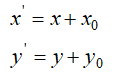我们对测试图进行水平和垂直正方向平移100像素，效果图如图Fig.1所示。

注意，黑色区域是我们默认填充的颜色，平移变换会出现图像跑到原图画布外面的情况，此时，原来的区域可以填充任意颜色，图像平移变换就这么简单。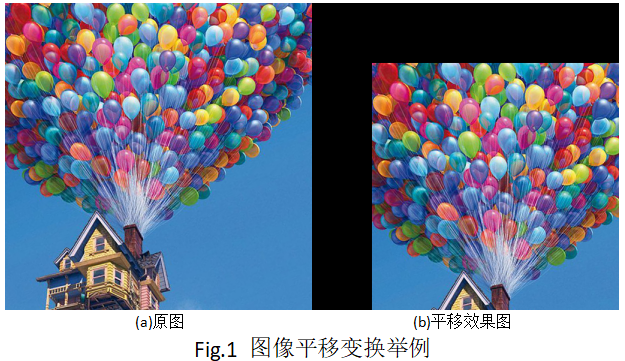[绘制与代码]

我们用C语言来实现图像平移变换算法，定义f_Transform.h文件，在文件中定义如下接口：

/*********************************************************
*Function:图像平移变换
*Params:
*          srcData:32bgra图像数据
*          width:图像宽度
*          height:图像高度
*          stride:图像幅度，对于32bgra格式而言，stride=width*4
*          xoffset：水平方向平移量
*          yoffset：垂直方向平移量
*Return:  0-成功，其他失败
*********************************************************/
int f_XYOfffset(unsigned char* srcData, int width, int height, int stride, int xoffset, int yofffset);

在这个接口中，定义了两个参数xoffset和yoffset分别用来表示水平和垂直的偏移向量，注意，如果xoffset和yoffset都为正数，则表示的是向水平和垂直的正方向偏移了xoffset和yoffset个像素距离，表现在结果图中，即图像向左偏移，反之，图像向右边偏移。

完整接口代码如下：

/*********************************************************
*Function:图像平移变换
*Params:
*          srcData:32bgra图像数据
*          width:图像宽度
*          height:图像高度
*          stride:图像幅度，对于32bgra格式而言，stride=width*4
*          xoffset：水平方向平移向量
*          yoffset：垂直方向平移向量
*Return:  0-成功，其他失败
*********************************************************/
int f_XYOfffset(unsigned char* srcData, int width, int height, int stride, int xoffset, int yoffset)
{
int ret = 0;
unsigned char* tempData = (unsigned char*)malloc(sizeof(unsigned char) * height * stride);
memcpy(tempData, srcData, sizeof(unsigned char) * height * stride);
unsigned char* pSrc = srcData;
for(int j = 0; j < height; j++)
{
for(int i = 0; i < width; i++)
{
int cx = i + xoffset;
int cy = j + yoffset;
if(cx >= 0 && cx < width && cy >= 0 && cy < height)
{
int pos = cx * 4 + cy * stride;
pSrc = tempData[pos];
pSrc = tempData[pos + 1];
pSrc = tempData[pos + 2];
}
else
{
pSrc = 0;
pSrc = 0;
pSrc = 0;
}
pSrc += 4;
}
}
free(tempData);
return ret;
};

接口到这里就写完了，仅仅30行左右，下面我们来写个测试代码：

#include "stdafx.h"
#include"imgRW\f_SF_ImgBase_RW.h"
#include"f_Transform.h"
int _tmain(int argc, _TCHAR* argv[])
{
//定义输入图像路径
char* inputImgPath = "Test.png";
//定义输出图像路径
char* outputImgPath = "res_offset.jpg";
//定义图像宽高信息
int width = 0, height = 0, component = 0, stride = 0;
//图像读取(得到32位bgra格式图像数据)
stride = width * 4;
int ret = 0;
//其他图像处理操作(这里以32位彩色图像灰度化为例)
//IMAGE PROCESS/
//图像平移
int xoffset = -100;//图像向右平移
int yoffset = -100;//图像向左平移
//调用图像平移变换接口
ret = f_XYOfffset(bgraData, width, height, stride,xoffset, yoffset);
//保存平移变换结果图
ret = Trent_ImgBase_ImageSave(outputImgPath, width, height, bgraData, JPG);
printf("f_XYOffset is finished!");

}
return 0;

可以看到，在这段代码中，我们使用的都是C语言标准库，对于初学者而言，非常方便，通俗易懂。

图像缩放变换

[定义与算法]

图像缩放即图像缩小与放大，是图像处理中最常用的操作，可以说图像处理离不开图像缩放，好的图像缩放算法可以高清还原图像信息，对于各种复杂的图像应用而言意义重大。

假设图像中任意一点(x,y)，按照水平方向缩放比例a和垂直方向缩放比例b进行缩放，则缩放后点坐标(x’,y’)的计算如下：

当a和b小于1时，表现为缩小，大于1时表现为图像放大，等于1时不缩放；既然有了放大与缩小，就存在图像信息的删除与填充，如何进行精确计算缩放后的坐标位置？这里引入一个必需要讲解的内容---图像插值算法。

图像插值算法有很多，从最邻近插值，到二次插值、三次插值、卷积插值等等以及超分辨率算法等高级插值，可以说，图像插值是一门学问，单独成书的也很多很多。这里，我们讲解两种最常用也是最基础的插值算法：最邻近插值和双线性二次插值。

最邻近插值不明思义就是用距离它最近的点来代替它。如下图Fig.2所示，有A和B两个像素点，A的值为100，B的值为20，要计算AB之间的C点像素值，其中，C点距离A的距离为0.4，距离B的距离为0.6，那么，C点距离A点像素最近，则C=100，这就是最邻近插值。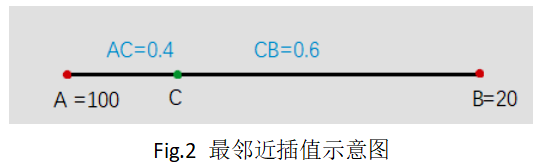最邻近插值计算量最小，但效果较差，往往会出现锯齿问题，即缩放后的图像边缘会出现锯齿毛刺，如图Fig.3所示，左边为原图，右边为最邻近插值放大两倍的结果，可以看到字母的边缘出现了锯齿状，非常不平滑。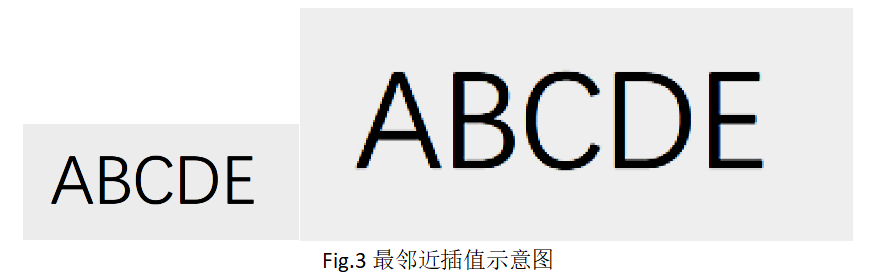双线性二次插值也称为一阶插值，这个算法说复杂也复杂，说简单也简单，如果你要从数学上讲明白，那要从拉格朗日插值多项式说起。

拉格朗日插值：

对于给定的n+1个节点，x0,x1,..xn，如果能够找到n+1个多项式，满足如下条件：，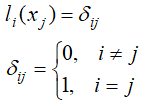那么，拉格朗日插值多项式P(x)表示如下：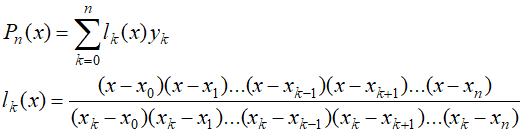其中，l(x)被称作插值基函数。

上述内容可以参考张铁所著《数值分析》136页部分，上述内容与二次插值有什么关系呢？这里我们一道例题来说明，该例题也来自张铁《数值分析》。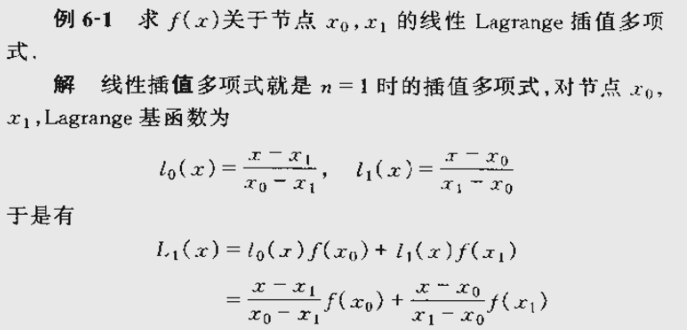对于x0和x1，它的一阶拉格朗日插值多项式L(x)求取如上所示，其中l0(x)和l1(x)分别表示对应权重，如果要计算x0到x1之间的任意一点x，那么计算L1(x)即可，看到这里我们明白，如果给定两个点，计算两点之间的任意一点插值，那么，我们只要可以使用上述1阶拉格朗日插值多项式即可，而这个方法就是二次插值的基础。

对于双线性二次插值，我们假设要计算的插值点(x,y)的值为f(x,y)，在它的附近有有f(i,j)，f(i+1,j)，f(i,j+1)和f(i+1,j+1)四个点，如下图Fig.4所示，f(x,y)的计算方法如下：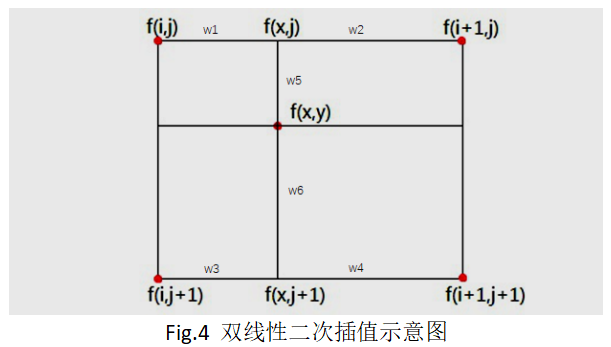①我们使用一阶拉格朗日插值计算点(i,j)和(i+1,j)之间的点(x,j)处的插值f(x,j)；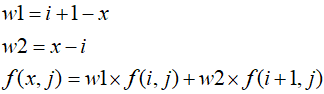②我们使用一阶拉格朗日插值计算点(i,j+1)和(i+1,j+1)之间的点(x,j+1)处的插值f(x,j+1)；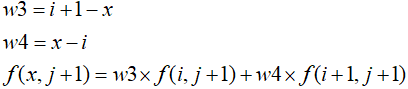③我们使用一阶拉格朗日插值计算(x,j)和(x,j+1)之间点(x,y)处的插值f(x,y)；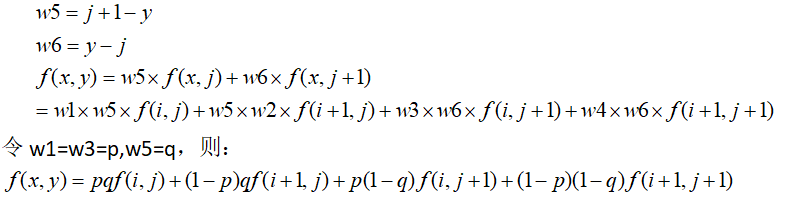这个公式就是双线性插值公式。我们用这个公式，对比最邻近插值效果，如下图Fig.5所示。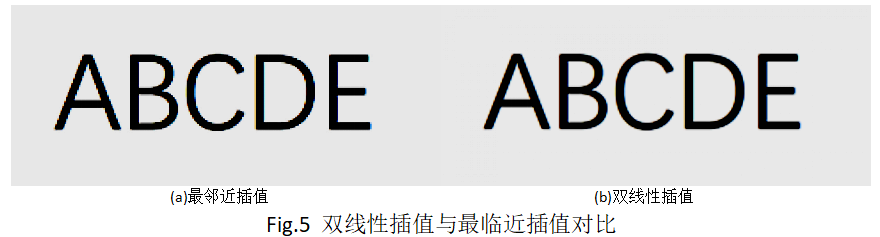[绘制与代码]

有了上述算法的解析，下面我们通过C语言来实现，首先定义接口如下：

/*********************************************************
*Function:图像缩放变换
*Params:
*          srcData:32bgra图像数据
*          width:图像宽度
*          height:图像高度
*          stride:图像幅度，对于32bgra格式而言，stride=width*4
*          scaleX:水平方向缩放比例,[0,]
*          scaleY:垂直方向缩放比例,[0,]
*          outW:缩放结果图宽度
*          outH:缩放结果图高度
*          outStride:缩放结果图Stride
*          interpolation：插值方式，0-最邻近插值，1-双线性插值
*Return:  缩放图像bgra数据指针
*********************************************************/
unsigned char* f_Zoom(unsigned char* srcData, int width, int height, int stride, float scaleX, float scaleY, int* outW, int* outH, int * outStride, int interpolation);

我们定义了f_Zoom的接口，这个接口中，由于缩放会改变图像大小，因此，我们返回一个缩放后的图像数据指针，同时，返回缩放后的图像宽高信息outW、outH和outStride；由于缩放包含水平和垂直方向的缩放因子，所以，添加水平缩放参数scaleX和垂直缩放参数scaleY，当scaleX小于1时表示水平缩小，等于1表示水平不缩放，大于1表示水平放大，垂直方向亦如此；最后，由于我们可以使用最邻近插值和双线性插值两种方式进行缩放，因此，添加了interpolation插值参数；

有了接口，我们给出完整的接口实现代码如下：

/*********************************************************
*Function:图像缩放变换
*Params:
*          srcData:32bgra图像数据
*          width:图像宽度
*          height:图像高度
*          stride:图像幅度，对于32bgra格式而言，stride=width*4
*          scaleX:水平方向缩放比例,[0,]
*          scaleY:垂直方向缩放比例,[0,]
*          outW:缩放结果图宽度
*          outH:缩放结果图高度
*          outStride:缩放结果图Stride
*          interpolation：插值方式，0-最邻近插值，1-双线性插值
*Return:  缩放图像bgra数据指针
*********************************************************/
unsigned char* f_Zoom(unsigned char* srcData, int width, int height, int stride, float scaleX, float scaleY, int* outW, int* outH, int * outStride, int interpolation)
{
int w = width * scaleX;
int h = height * scaleY;
int s = w * 4;
unsigned char* tempData = (unsigned char*)malloc(sizeof(unsigned char) * s * h);
memset(tempData, 255, sizeof(unsigned char) * s * h);
unsigned char* pTemp = tempData;
//最邻近插值
if(interpolation == 0)
{
for(int j = 0; j < h; j++)
{
for(int i = 0; i < w; i++)
{
int cx = CLIP3(i * width / w, 0, width - 1);
int cy = CLIP3(j * height / h, 0, height - 1);
int pos = cx * 4 + cy * stride;
pTemp = srcData[pos];
pTemp = srcData[pos + 1];
pTemp = srcData[pos + 2];
pTemp = srcData[pos + 3];
pTemp += 4;
}
}
}
else//双线性插值
{
for(int j = 0; j < h; j++)
{
for(int i = 0; i < w; i++)
{
float cx = CLIP3((float)i * width / w, 1, width - 2);
float cy = CLIP3((float)j * height / h, 1, height - 2);
int tx = (int)cx;
int ty = (int)cy;
float p = abs(cx - tx);
float q = abs(cy - ty);
int pos = tx * 4 + ty * stride;
int p1 = pos;
int p2 = pos + stride;
int p3 = pos + 4;
int p4 = pos + 4 + stride;
float a = (1.0f - p) * (1.0f - q);
float b = (1.0f - p) * q;
float c = p * (1.0f - q);
float d = p * q;
pTemp = CLIP3((a * srcData[p1 + 0] + b * srcData[p2 + 0] + c * srcData[p3 + 0] + d * srcData[p4 + 0]), 0, 255);
pTemp = CLIP3((a * srcData[p1 + 1] + b * srcData[p2 + 1] + c * srcData[p3 + 1] + d * srcData[p4 + 1]), 0, 255);
pTemp = CLIP3((a * srcData[p1 + 2] + b * srcData[p2 + 2] + c * srcData[p3 + 2] + d * srcData[p4 + 2]), 0, 255);
pTemp = CLIP3((a * srcData[p1 + 3] + b * srcData[p2 + 3] + c * srcData[p3 + 3] + d * srcData[p4 + 3]), 0, 255);
pTemp += 4;
}
}
}
*outW = w;
*outH = h;
*outStride = s;
return tempData;
};

代码不足60行，通俗易懂，最后给出接口的调用代码：

#include "stdafx.h"
#include"imgRW\f_SF_ImgBase_RW.h"
#include"f_Transform.h"
int _tmain(int argc, _TCHAR* argv[])
{
//定义输入图像路径
char* inputImgPath = "t150.png";
//定义图像宽高信息
int width = 0, height = 0, component = 0, stride = 0;
//图像读取(得到32位bgra格式图像数据)
stride = width * 4;
int ret = 0;
//其他图像处理操作(这里以32位彩色图像为例)
//IMAGE PROCESS/
//图像缩放
int outW, outH, outS;
float scaleX = 5;
float scaleY = 5;
int interpolation = INTERPOLATE_BILINEAR;//INTERPOLATE_NEAREST;
char* outZoomImgPath = "res_zoom.png";
unsigned char* pResZoom = f_Zoom(bgraData, width, height, stride, scaleX, scaleY, &outW, &outH, &outS, interpolation);
ret = Trent_ImgBase_ImageSave(outZoomImgPath, outW, outH, pResZoom, PNG);
free(pResZoom);
printf("f_zoom is finished!");

return 0;
}

效果测试如下图Fig.6所示：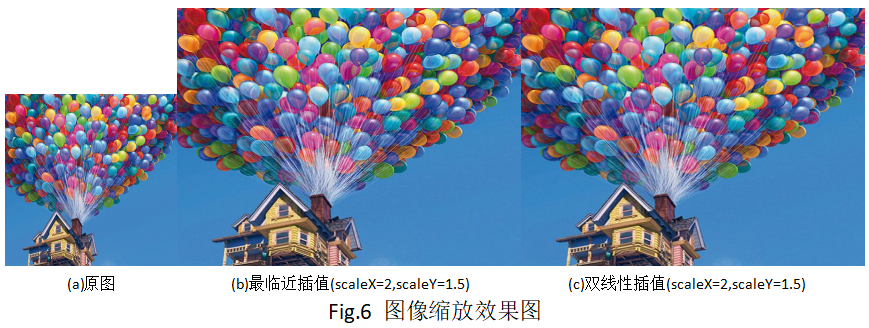图像旋转变换

[定义与算法]

图像旋转即将图像按照某个原点顺时针或者逆时针旋转某个角度。

如果平面上所有点(x,y)绕原点O旋转角度，旋转后的点为(x’,y’)，则两者正向和逆向计算公式如下：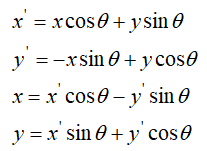公式的推导我们以正向变换为例，在极坐标系中，假设(x,y)到原点O距离为r，注意，这里O点表示上文平移后的图像中心点，(x,y)和原点的连线与x轴方向的夹角为b，旋转角度为a，旋转后坐标为(x’,y’)，如下图Fig.7所示，则按照极坐标公式有：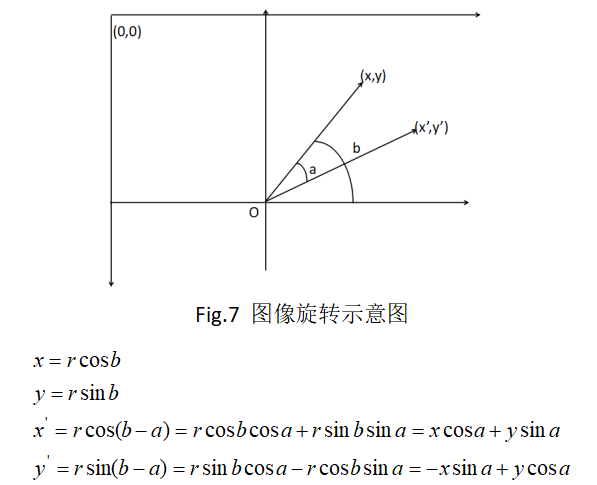上述便是正向推导过程，即由(x,y)到旋转后的点(x’,y’)。

在实际中，图像平面中的原点一般为左上角，也就是左上角为(0,0)点，垂直方向向下为正方向，与正常的笛卡尔坐标系不同。我们想要的往往是图像围绕图像中心点进行角度旋转，这个时候，我们需要把点(x,y)先转换为以图像中心为原点的坐标，也就是进行一定的坐标平移，然后再进行旋转变换。同时，为例避免孔洞现象，我们一般在计算的过程中，是按照逆向变换，根据目标图像素位置(x’,y’)计算它在原图中的位置(x,y)，一次完成旋转变换的。

假设旋转后图像的宽为W’，高为H’，点(x’,y’)映射到原图中的坐标为(x,y)，则计算过程如下：

①按照平移逆变换将(x’,y’)进行平移：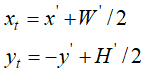②按照旋转逆变换公式将(x,y)进行变换：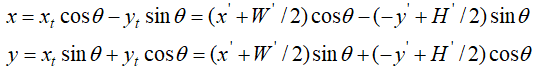③将坐标原点由图像中心平移至左上角：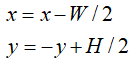④根据(x,y)位置选择插值算法插值得到最终旋转后的像素值；

上面的过程就是完整的图像旋转变换，下面我们将动手实践一下。

[绘制与代码]

我们首先定义一个图像旋转的接口，如下：

/*********************************************************
*Function:图像旋转变换
*Params:
*          srcData:32bgra图像数据
*          width:图像宽度
*          height:图像高度
*          stride:图像幅度，对于32bgra格式而言，stride=width*4
*          angle:图像旋转角度
*          outW:旋转结果图宽度
*          outH:旋转结果图高度
*          outStride:旋转结果图Stride
*          interpolation：插值方式，0-最邻近插值，1-双线性插值
*Return:  旋转图像bgra数据指针
*********************************************************/
unsigned char* f_Rotate(unsigned char* srcData, int width, int height, int stride, int angle, int* outW, int* outH, int* outStride, int interpolation);

图像旋转变换中，图像的大小发生了变化，因此这里我们的接口返回一个变换后的图像数据指针，与缩放接口类似，添加新图像宽高输出参数outW，outH和outStride；由于旋转变换需要角度信息，因此这里添加了角度输入参数angle，范围为0到360度，同时，设置插值算法参数interpolation；

按照前文的旋转变换算法公式，我们给出C代码如下：

/*********************************************************
*Function:图像旋转变换
*Params:
*          srcData:32bgra图像数据
*          width:图像宽度
*          height:图像高度
*          stride:图像幅度，对于32bgra格式而言，stride=width*4
*          angle:图像旋转角度
*          outW:旋转结果图宽度
*          outH:旋转结果图高度
*          outStride:旋转结果图Stride
*          interpolation：插值方式，0-最邻近插值，1-双线性插值
*Return:  旋转图像bgra数据指针
*********************************************************/
unsigned char* f_Rotate(unsigned char* srcData, int width, int height, int stride, int angle, int* outW, int* outH, int* outStride, int interpolation)
{
float degree = angle * PI / 180.0f;
float cx = 0, cy = 0, Cos = 0, Sin = 0;
Cos = cos(degree);
Sin = sin(degree);
//计算新图像的宽高
int w = width * Cos + height * Sin;
int h = height * Cos + width * Sin;
int s = w * 4;
*outW = w;
*outH = h;
*outStride = s;
//常量计算，用来优化速度
cx = -w / 2.0f * Cos - h / 2.0f * Sin + width / 2.0f;
cy = w / 2.0f * Sin - h / 2.0f * Cos + height / 2.0f;
unsigned char* tempData = (unsigned char*)malloc(sizeof(unsigned char) * s * h);
memset(tempData, 255, sizeof(unsigned char) * s * h);
unsigned char* pTemp = tempData;
//最邻近插值
if(interpolation == 0)
{
for(int j = 0; j < h; j++)
{
for(int i = 0; i < w; i++)
{
//这里实际上就是按照公式计算，进行了优化，把一些常量计算放到了外面的cx和cy中
int tx = i * Cos + j * Sin + cx;
int ty = j * Cos - i * Sin + cy;
if(tx >= 0 && tx < width && ty >= 0 && ty < height)
{
int pos = tx * 4 + ty * stride;
pTemp = srcData[pos];
pTemp = srcData[pos + 1];
pTemp = srcData[pos + 2];
pTemp = srcData[pos + 3];
}
else
{
pTemp = 0;
pTemp = 0;
pTemp = 0;
pTemp = 255;
}
pTemp += 4;
}
}
}
else//双线性插值
{
for(int j = 0; j < h; j++)
{
for(int i = 0; i < w; i++)
{
//这里实际上就是按照公式计算，进行了优化，把一些常量计算放到了外面的cx和cy中
float mx = i * Cos + j * Sin + cx;
float my = j * Cos - i * Sin + cy;
if(mx >= 0 && mx < width && my >= 0 && my < height)
{
int tx = (int)mx;
int ty = (int)my;
float p = abs(mx - tx);
float q = abs(my - ty);
int pos = tx * 4 + ty * stride;
int p1 = pos;
int p2 = pos + stride;
int p3 = pos + 4;
int p4 = pos + 4 + stride;
float a = (1.0f - p) * (1.0f - q);
float b = (1.0f - p) * q;
float c = p * (1.0f - q);
float d = p * q;
pTemp = CLIP3((a * srcData[p1 + 0] + b * srcData[p2 + 0] + c * srcData[p3 + 0] + d * srcData[p4 + 0]), 0, 255);
pTemp = CLIP3((a * srcData[p1 + 1] + b * srcData[p2 + 1] + c * srcData[p3 + 1] + d * srcData[p4 + 1]), 0, 255);
pTemp = CLIP3((a * srcData[p1 + 2] + b * srcData[p2 + 2] + c * srcData[p3 + 2] + d * srcData[p4 + 2]), 0, 255);
pTemp = CLIP3((a * srcData[p1 + 3] + b * srcData[p2 + 3] + c * srcData[p3 + 3] + d * srcData[p4 + 3]), 0, 255);
}
else
{
pTemp = 0;
pTemp = 0;
pTemp = 0;
pTemp = 255;
}
pTemp += 4;
}
}
}
return tempData;
};

本文的代码可以看到，基本都是将插值的interpolation条件判断放到了循环外面，导致代码段较长，实际上这样做是为了优化速度，增强代码可读性，大家可以体会一下。

我们对上面接口进行调用测试如下：

#include "stdafx.h"
#include"imgRW\f_SF_ImgBase_RW.h"
#include"f_Transform.h"
int _tmain(int argc, _TCHAR* argv[])
{
//定义输入图像路径
char* inputImgPath = "Test.png";
//定义图像宽高信息
int width = 0, height = 0, component = 0, stride = 0;
//图像读取(得到32位bgra格式图像数据)
stride = width * 4;
int ret = 0;
//其他图像处理操作(这里以32位彩色图像为例)
//IMAGE PROCESS/
//图像旋转
int outW, outH, outS;
int angle = 80;
int interpolation = INTERPOLATE_NEAREST;//INTERPOLATE_BILINEAR;//INTERPOLATE_NEAREST;
char* outRotateImgPath = "res_rotate_nearest.jpg";
unsigned char* pResRotate = f_Rotate(bgraData, width, height, stride, angle, &outW, &outH, &outS, interpolation);
ret = Trent_ImgBase_ImageSave(outRotateImgPath, outW, outH, pResRotate, JPG);
free(pResRotate);
printf("f_Rotate is finished!");

return 0;
}

大家可以看到，调用非常方便，甚至比opencv更加通俗易懂。最后我们给出对应的测试效果，如图Fig.8所示。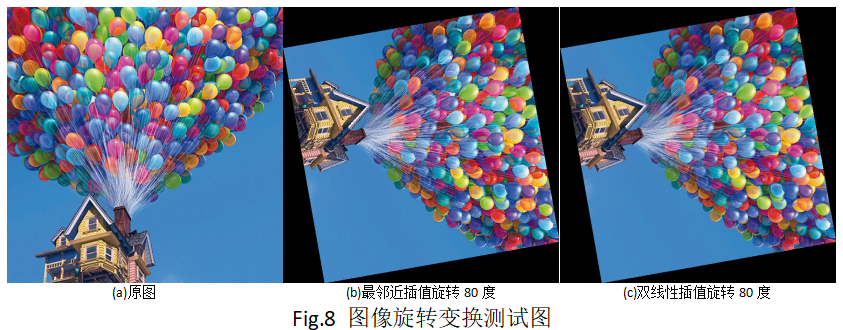本节完整的代码工程关注本人公众号“SF图像算法”有相关下载链接即可免费下载。

[知识扩展]

在本文中，我们详细了解了图像平移、图像缩放和图像旋转三种图像几何变换，也是最常用的图像基本变换。在这个过程中我们是单个一一讲解的，为的是让新手同学们能够各个击破，单独理解。而实际中，这三个变换可以通过一个仿射变换矩阵来统一表达，放这边还矩阵如下：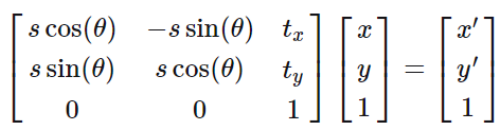其中，s表示缩放比例，tx和ty表示平移量，theta表示角度；

按照这个公式，我们可以一次计算出三种变换后对应的结果(x’,y’)，然后再进行插值计算即可，大家可以自己尝试一下；

对于图像变形和插值算法，多少年来，有无数相关的论文研究，近几年来，随着深度学习的飞速发展，基于卷积神经网络的超分辨率，图像复原以及图像变形的算法也是层出不穷，可见其意义之大！

最后，学海无涯，共勉！


展开全文图像旋转 图像缩放
• 本系列python版本：python3.5.4 本系列opencv-python版本：opencv-python3.4.2.17 本系列使用的开发环境是jupyter notebook，是一个python的交互式开发环境，测试十分方便，并集成了...上文【数字图像处理系列二...线性变换 分段线性变换 对数变换 幂律变换 直方图均衡化
• 实验内容及实验原理：1、灰度的线性变换灰度的线性变换就是将图像中所有的点的灰度按照线性灰度变换函数进行变换。该线性灰度变换函数是一个一维线性函数：f(x)=a*x+b其中参数a为线性函数的斜率，b为线性函数的在y轴...opencv 图像
• 前些天本来打算用VC6.0 + opencv1.0去学习图像处理，但后来发现还是VS + opencv2以上...因为作业的要求，所以写了实现了灰度图像的直方均衡、线性变换与线性拉伸的程序。如果对上述几个概念不是很清楚可以参考下这篇opencv vs2015 直方图均衡
• 图像处理开发资料、图像处理开发需求、图像处理接私活挣零花钱，可以搜索公众号"qxsf321"，并关注！ 图像处理开发资料、图像处理开发需求、图像处理接私活挣零花钱，可以搜索公众号"qxsf321"，...
• 使用C++、opencv实现对图像的对数变换及非线性变换，实现图像增强 相关API： void normalize(InputArray src, OutputArray dst, double alpha=1, double beta=0, int norm_type=NORM_L2, int dtype=-1, Input...图像增强 对数变换 非线性变换 normalize
• 图像的灰度线性变换图像灰度变换的一种，图像的灰度变换通过建立灰度映射来调整源图像的灰度，从而达到图像增强的目的。灰度映射通常是用灰度变换曲线来进行表示。通常来说，它是将图像的像素值通过指定的线性函数...
• 软件实习 利用OpenCV开源库，实现一个图像处理程序，可实现图像的读，写功能，简单的图像平滑，锐化，线性变换等功能。 说明：请自行配制Opne cv 1.0 否则软件运行在VC++ 6.0会报错！
• 图像的灰度线性变换图像灰度变换的一种，图像的灰度变换通过建立灰度映射来调整源图像的灰度，从而达到图像增强的目的。灰度映射通常是用灰度变换曲线来进行表示。通常来说，它是将图像的像素值通过指定的线性函数...
• 图像的灰度变换处理是图像增强处理技术中的一种非常基础、直接的空间域图像处理方法，也是图像数字化软件和图像显示软件的一个重要组成部分。 采用灰度变换法对图像进行处理可以大大改善图像的视觉效果。灰度变换...机器学习 图像处理
• 线性灰度变换函数f(x)是一个一维线性函数 y=f(x)=ax+b 式中；a为线性函数的斜率，b为线性函数在y轴的截距，x表示输入图像的灰度值，y表示输出图像的灰度值。 （1） 当a>1时，输出图像的对比度将增加；当a ...
• // 对比度增强.cpp : 定义控制台应用程序的入口点。 // #include "stdafx.h" #include #include #include using namespace cv; using namespace std; //归一化 //data 进行处理的像素集合 //grayscale ...
• （2）将图像转换为一种更适合人或机器进行分析处理的形式，抑制无用信息，提高图像使用价值。 图像增强从作用域划分为：空间域增强、频率域增强、彩色增强。 其中，空间域增强是直接对图像像素灰度进行操作。包括...matlab
• 而在图像处理中也有傅里叶分析的概念，我这里给出在其官方指导文件opencv_tutorials中给出的解释。  傅里叶变换可以将一幅图片分解为正弦和余弦两个分量，换而言之，他可以将一幅图像从其空间域图像处理 opencv 算法
• 基于MATLAB的数字图像处理———灰度变换与空间滤波 对于图像平面，定义为二维函数 f (x,y),其中x,y分别为空间横竖坐标。当f,x,y都是有限离散值时，图像即为数字图像。 一、图像的输入/输出和显示 相关函数： ...
• 概念在图像处理中的空间变换（spatial transformation）分成两种情况，有仿射变换（Affine Transformation）及投影变换（Perspective Transformation）。仿射变换是从一个二维坐标变换到另一个二维坐标，它是一种...图像处理 空间变换 投影变换 变换矩阵
...